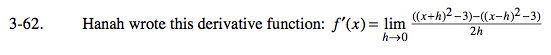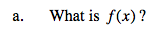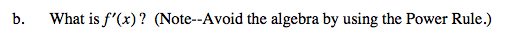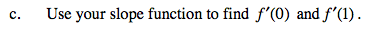### Home > CALC > Chapter Ch3 > Lesson 3.2.2 > Problem3-62

3-62.Hanah's (symmetrical difference) definition of the derivative;

$f'(x)=\lim_{h\rightarrow 0}\frac{f(x+h)-f(x-h)}{2h}$

f(x) = x2 − 3

Find f '(x), which is also the value of the limit.Power Rule
if f(x) = xn
then f '(x) = nxn − 1

f(x) = x2 − 3
f '(x) = __________Evaluate.